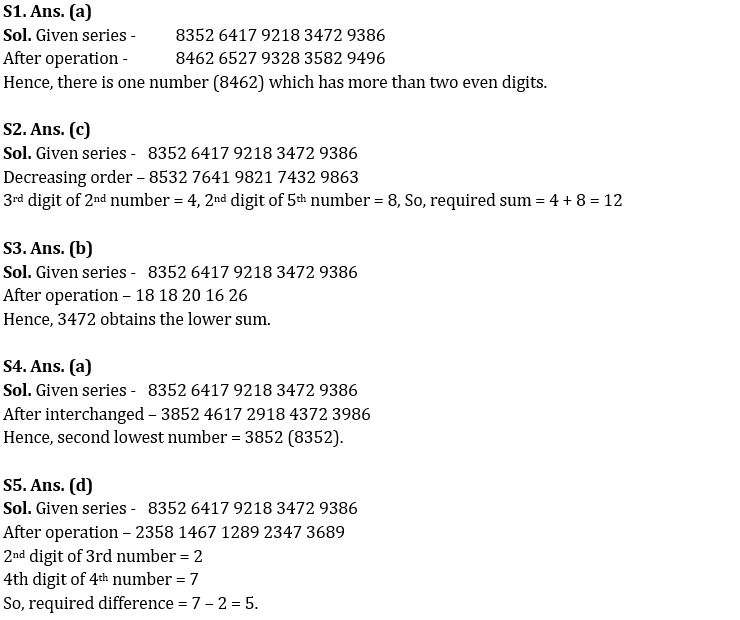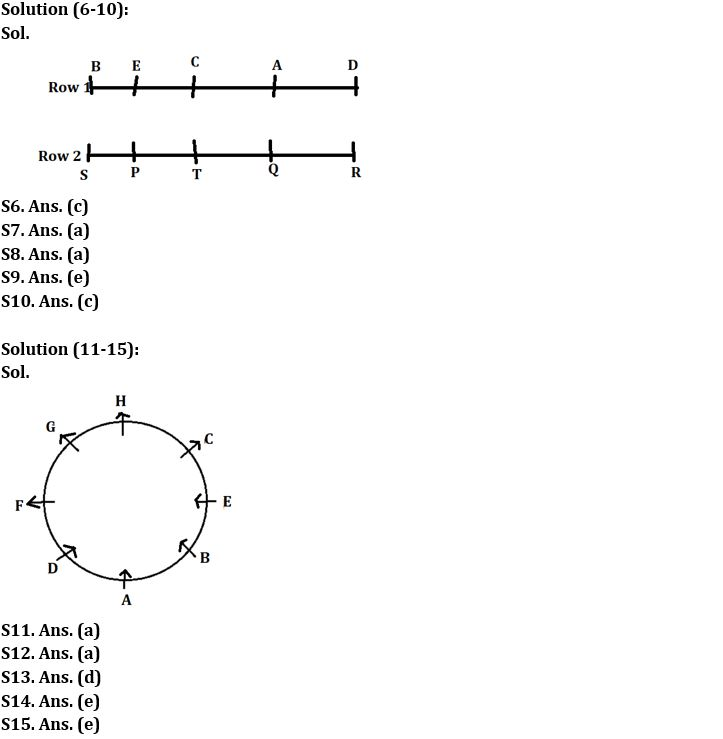Latest Banking jobs   »

# Reasoning Ability Quiz For IBPS RRB PO Clerk Prelims 2022- 11th August

Directions (1-5): Answer the questions based on the information given below.

8352   6417   9218   3472   9386

Note: The 1st, 2nd, 3rd and so on numbers or digits or elements are taken from left end.

Q1. If 1 is added to 2nd and 3rd digits of all the given numbers, then how many numbers will have more than two even digits?
(a) One
(b) Two
(c) Three
(d) More than three
(e) None of these

Q2. If digits of all the given numbers are arranged in decreasing order, then what will be the sum of 3rd digit of 2nd number and 2nd digit of 5th number?
(a) 11
(b) 13
(c) 12
(d) 15
(e) None of these

Q3. If digits of all the numbers are added, then which number obtains the lower sum?
(a) 8352
(b) 3472
(c) 9218
(d) 9386
(e) None of these

Q4. If 1nd and 2nd digit of each number is interchanged then which number will be the second lowest?
(a) 8352
(b) 3472
(c) 9218
(d) 9386
(e) 6417

Q5. If digits of all the given numbers are arranged in ascending order, then what will be the difference of 2nd digit of 3rd number and 4th digit of 4th number?
(a) 10
(b) 6
(c) 8
(d) 5
(e) None of these

Directions (6-10): answer the questions based on the information given below.
Five persons A, B, C, D and E are sitting in row 1 facing south whereas P, Q, R, S and T are sitting in row 2 facing north such that both rows are parallel and equidistant from each other but not necessarily in the same order. Row 2 is in south of Row 1.
Two persons sits between E and D who sits opposite to R. D sits to the left of A. S does not sit opposite of C. Two persons sit between A and B who sits at one of the extreme ends of the row. A sits opposite to Q. One person sits between Q and P. R does not sit adjacent to P.

Q6. Who among the following person sits second to the right of C?
(a) A
(b) D
(c) B
(d) E
(e) None of these

Q7. How many persons sit between A and E?
(a) One
(b) Two
(c) Three
(d) Either one or two
(e) None of these

Q8. What is the position of Q with respect to R?
(a) Immediate left
(b) Second to the left
(c) Immediate right
(d) Third to the right
(e) None of these

Q9. Four from the following are similar in a certain way and forms a group. Find out the one, which does not belong to that group.
(a) A, Q
(b) B, S
(c) E, P
(d) D, R
(e) T, B

Q10. Who among the following sits 3rd to the right of S?
(a) P
(b) T
(c) Q
(d) R
(e) Can’t be determined

Directions (11-15): answer the questions based on the information given below.
Eight persons A, B, C, D, E, F, G and H are sitting around a circular table such that some of them are facing towards the center while some of them face away from the center but not necessarily in the same order.
C sits 3rd to the right of A who sits 3rd to the left of G. Three persons sit between C and D. E sits 3rd to the right of D but not adjacent to G. Both C and H face same direction as G. E face same direction as B. F sits 3rd to the left of B who does not sit adjacent to G. F does not sit adjacent to C. H sits 2nd to the right of F who faces away from the center.

Q11. Who sits 3rd to the right of H?
(a) B
(b) A
(c) F
(d) D
(e) None of these

Q12. Who sits 2nd to the right of E?
(a) H
(b) A
(c) F
(d) B
(e) None of these

Q13. How many persons sit between E and A when counted from left of A?
(a) One
(b) Two
(c) Three
(d) More than three
(e) None of these

Q14. What is the position of D with respect of F?
(a) 3rd to the right
(b) 2nd to the left
(c) Immediate right
(d) 4th to the left
(e) Immediate left

Q15. Four from the following are similar in a certain way and forms a group. Find out the one, which does not belong to that group.
(a) A
(b) B
(c) D
(d) E
(e) F

Solutions#### Congratulations!Download Hindu Review of October 2021: Free PDF